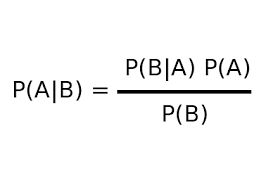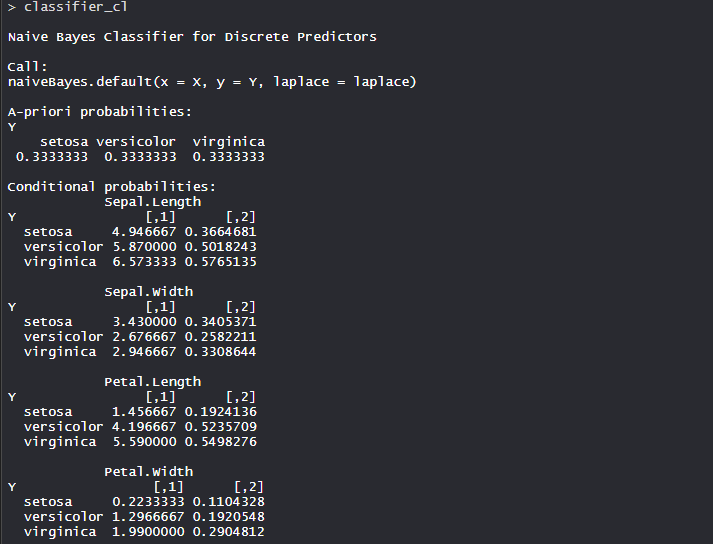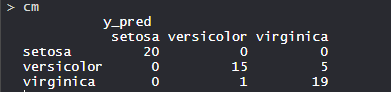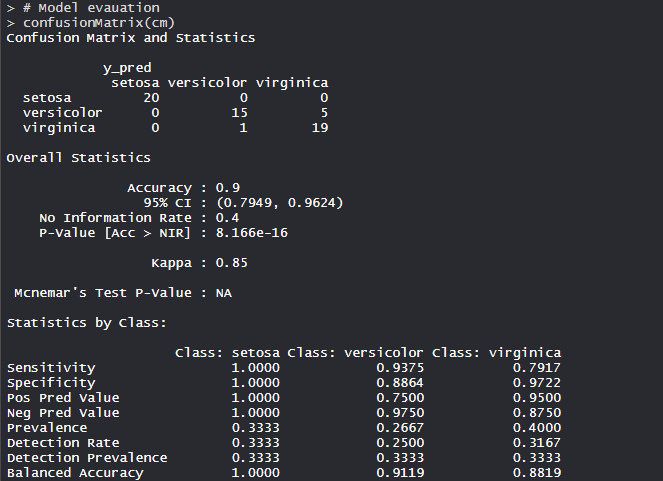Related Articles
Naive Bayes Classifier in R Programming
• Last Updated : 22 Jun, 2020

Naive Bayes is a Supervised Non-linear classification algorithm in R Programming. Naive Bayes classifiers are a family of simple probabilistic classifiers based on applying Baye’s theorem with strong(Naive) independence assumptions between the features or variables. The Naive Bayes algorithm is called “Naive” because it makes the assumption that the occurrence of a certain feature is independent of the occurrence of other features.

#### Theory

Naive Bayes algorithm is based on Bayes theorem. Bayes theorem gives the conditional probability of an event A given another event B has occurred.where,
P(A|B) = Conditional probability of A given B.
P(B|A) = Conditional probability of B given A.
P(A) = Probability of event A.
P(B) = Probability of event B.

For many predictors, we can formulate the posterior probability as follows:

P(A|B) = P(B1|A) * P(B2|A) * P(B3|A) * P(B4|A) …

Example

```Consider a sample space:
{HH, HT, TH, TT}
where,
T: Tail

P(Second coin being head given  = P(A|B)
first coin is tail) = P(A|B)
= [P(B|A) * P(A)] / P(B)
= [P(First coin is tail given second coin is head) *
P(Second coin being Head)] / P(first coin being tail)
= [(1/2) * (1/2)] / (1/2)
= (1/2)
= 0.5```

#### The Dataset

`Iris` dataset consists of 50 samples from each of 3 species of Iris(Iris setosa, Iris virginica, Iris versicolor) and a multivariate dataset introduced by British statistician and biologist Ronald Fisher in his 1936 paper The use of multiple measurements in taxonomic problems. Four features were measured from each sample i.e length and width of the sepals and petals and based on the combination of these four features, Fisher developed a linear discriminant model to distinguish the species from each other.

 `# Loading data``data(iris)``  ` `# Structure ``str``(iris)`#### Performing Naive Bayes on Dataset

Using Naive Bayes algorithm on the dataset which includes 11 persons and 6 variables or attributes

 `# Installing Packages``install.packages(``"e1071"``)``install.packages(``"caTools"``)``install.packages(``"caret"``)`` ` `# Loading package``library(e1071)``library(caTools)``library(caret)`` ` `# Splitting data into train``# and test data``split <``-` `sample.split(iris, SplitRatio ``=` `0.7``)``train_cl <``-` `subset(iris, split ``=``=` `"TRUE"``)``test_cl <``-` `subset(iris, split ``=``=` `"FALSE"``)`` ` `# Feature Scaling``train_scale <``-` `scale(train_cl[, ``1``:``4``])``test_scale <``-` `scale(test_cl[, ``1``:``4``])`` ` `# Fitting Naive Bayes Model ``# to training dataset``set``.seed(``120``)  ``# Setting Seed``classifier_cl <``-` `naiveBayes(Species ~ ., data ``=` `train_cl)``classifier_cl`` ` `# Predicting on test data'``y_pred <``-` `predict(classifier_cl, newdata ``=` `test_cl)`` ` `# Confusion Matrix``cm <``-` `table(test_cl\$Species, y_pred)``cm`` ` `# Model Evauation``confusionMatrix(cm)`

#### Output:

• Model classifier_cl:The Conditional probability for each feature or variable is created by model separately. The apriori probabilities are also calculated which indicates the distribution of our data.

• Confusion Matrix:So, 20 Setosa are correctly classified as Setosa. Out of 16 Versicolor, 15 Versicolor are correctly classified as Versicolor, and 1 are classified as virginica. Out of 24 virginica, 19 virginica are correctly classified as virginica and 5 are classified as Versicolor.

• Model Evaluation:The model achieved 90% accuracy with a p-value of less than 1. With Sensitivity, Specificity, and Balanced accuracy, the model build is good.

So, Naive Bayes is widely used in Sentiment analysis, document categorization, Email spam filtering etc in industry.

My Personal Notes arrow_drop_up